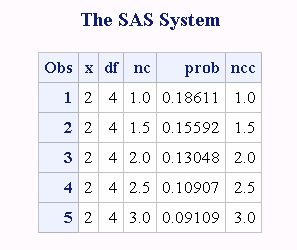# CNONCT Function

Returns the noncentrality parameter from a chi-square distribution.

 Category: Mathematical

## Syntax

CNONCT(x,df,prob)

### Required Arguments

#### x

is a numeric random variable.

 Range x ≥ 0

#### df

is a numeric degrees of freedom parameter.

 Range df > 0

#### prob

is a probability.

 Range 0 < prob < 1

## Details

The CNONCT function returns the nonnegative noncentrality parameter from a noncentral chi-square distribution whose parameters are x, df, and nc. If prob is greater than the probability from the central chi-square distribution with the parameters x and df, a root to this problem does not exist. In this case a missing value is returned. A Newton-type algorithm is used to find a nonnegative root nc of the equation
$equation$
The following relationship applies to the preceding equation:
$equation$
The following relationship applies to the preceding equation:
$equation$
is the probability from the gamma distribution given by
$equation$
If the algorithm fails to converge to a fixed point, a missing value is returned.

## Example

```data work;
x=2;
df=4;
do nc=1 to 3 by .5;
prob=probchi(x,df,nc);
ncc=cnonct(x,df,prob);
output;
end;
run;
proc print;
run;```
Computations of the Noncentrality Parameters from the Chi-squared Distribution